# Probability of Backtest Overfitting

The package pbo provides convenient functions for analyzing a matrix of backtest trials to compute the probability of backtest overfitting, the performance degradation, and the stochastic dominance of the fitted models. The approach follows that described by Bailey et al. in their paper “The Probability of Backtest Overfitting” (reference provided below).

First, we assemble the trials into an NxT matrix where each column represents a trial and each trial has the same length T. This example is random data so the backtest should be overfit.

set.seed(765)
n <- 100
t <- 2400
m <- data.frame(matrix(rnorm(n*t),nrow=t,ncol=n,dimnames=list(1:t,1:n)),
check.names=FALSE)

sr_base <- 0
mu_base <- sr_base/(252.0)
sigma_base <- 1.00/(252.0)**0.5

for ( i in 1:n ) {
m[,i] = m[,i] * sigma_base / sd(m[,i]) # re-scale
m[,i] = m[,i] + mu_base - mean(m[,i]) # re-center
}


We can use any performance evaluation function that can work with the reassembled sub-matrices during the cross validation iterations. Following the original paper we can use the Sharpe ratio as

sharpe <- function(x,rf=0.03/252) {
sr <- apply(x,2,function(col) {
er = col - rf
return(mean(er)/sd(er))
})
return(sr)
}


Now that we have the trials matrix we can pass it to the pbo function for analysis. The analysis returns an object of class pbo that contains a list of the interesting results. For the Sharpe ratio the interesting performance threshold is 0 (the default of 0) so we pass threshold=0 through the pbo call argument list.

require(pbo)

## Loading required package: pbo
## Loading required package: lattice

my_pbo <- pbo(m,s=8,f=sharpe,threshold=0)


The my_pbo object is a list we can summarize with the summary function.

summary(my_pbo)

## Performance function sharpe with threshold 0

##      p_bo     slope      ar^2    p_loss
##  1.000000 -0.003046  0.970000  1.000000


We see that the backtest overfitting probably is 1 as expected because all of the trials have the same performance. We can view the results with the package's preconfigured lattice plots. The xyplot function has several variations for the plotType parameter value. See the ?xyplot.pbo help page for the details.

require(lattice)
require(latticeExtra)

## Loading required package: latticeExtra
## Loading required package: RColorBrewer

require(grid)

## Loading required package: grid

histogram(my_pbo,type="density")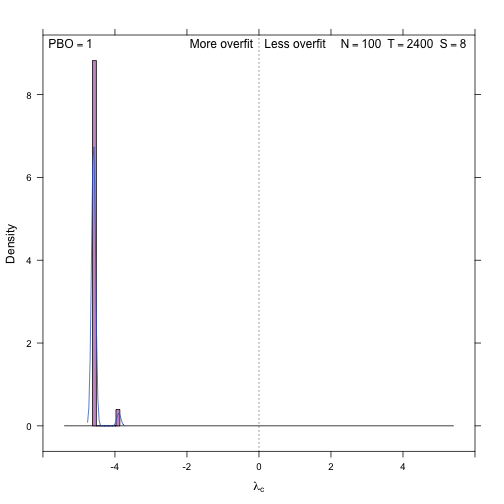xyplot(my_pbo,plotType="degradation")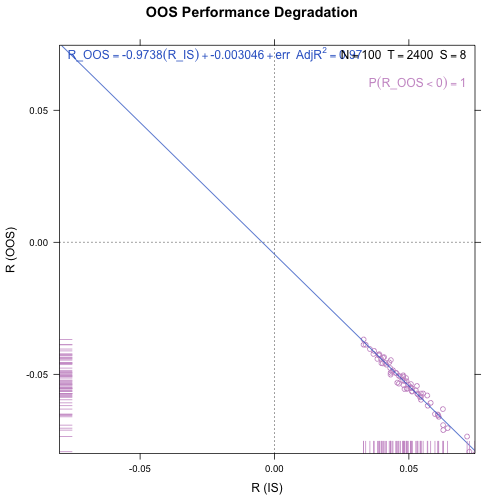xyplot(my_pbo,plotType="dominance",increment=0.001)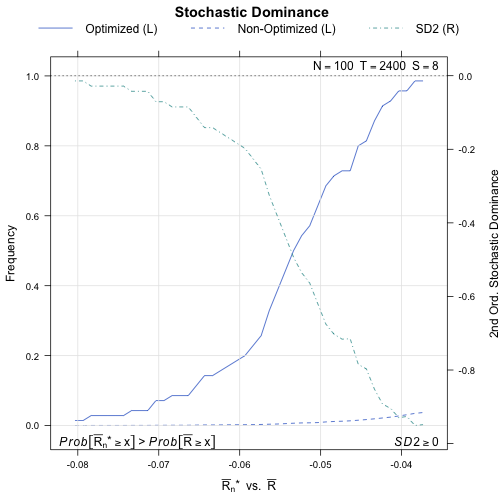xyplot(my_pbo,plotType="pairs")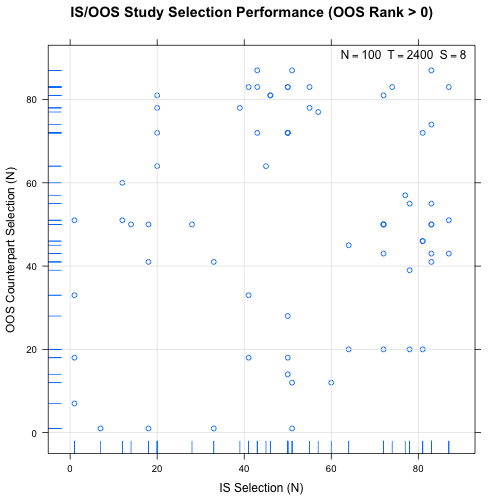xyplot(my_pbo,plotType="ranks",ylim=c(0,20))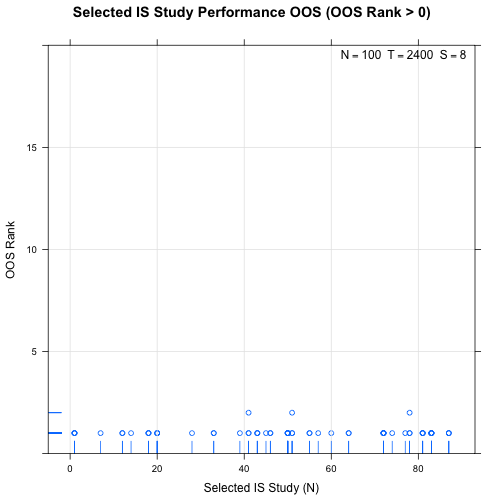dotplot(my_pbo)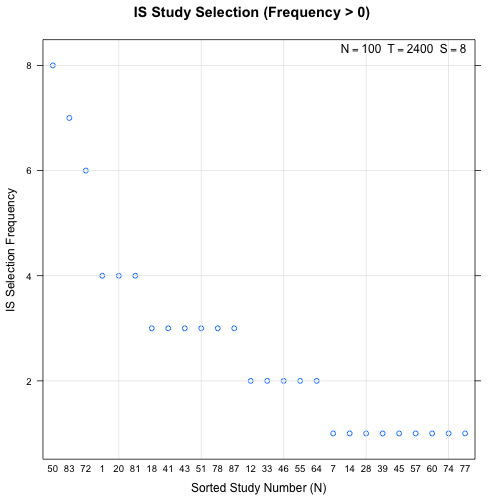The package also supports parallel execution on multicore hardware, providing a potentially significant reduction in pbo analysis time. The pbo package uses the foreach package to manage parallel workers, so we can use any package that supports parallelism using foreach.

For example, using the doParallel package we can establish a multicore cluster and enable multiple workers by passing the above m and s values along with the argument allow_parallel=TRUE to pboas follows:

require(doParallel)

## Loading required package: doParallel
## Loading required package: foreach
## Loading required package: iterators
## Loading required package: parallel

cluster <- makeCluster(2) # or use detectCores()
registerDoParallel(cluster)
p_pbo <- pbo(m,s=8,f=sharpe,allow_parallel=TRUE)
stopCluster(cluster)

summary(p_pbo)

## Performance function sharpe with threshold 0

##      p_bo     slope      ar^2    p_loss
##  1.000000 -0.003046  0.970000  1.000000


## Reference

Bailey, David H. and Borwein, Jonathan M. and Lopez de Prado, Marcos and Zhu, Qiji Jim, “The Probability of Back-Test Overfitting” (September 1, 2013). Available at SSRN.